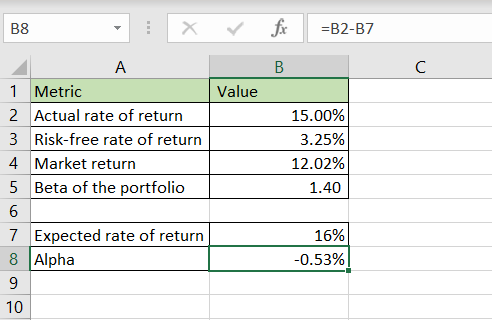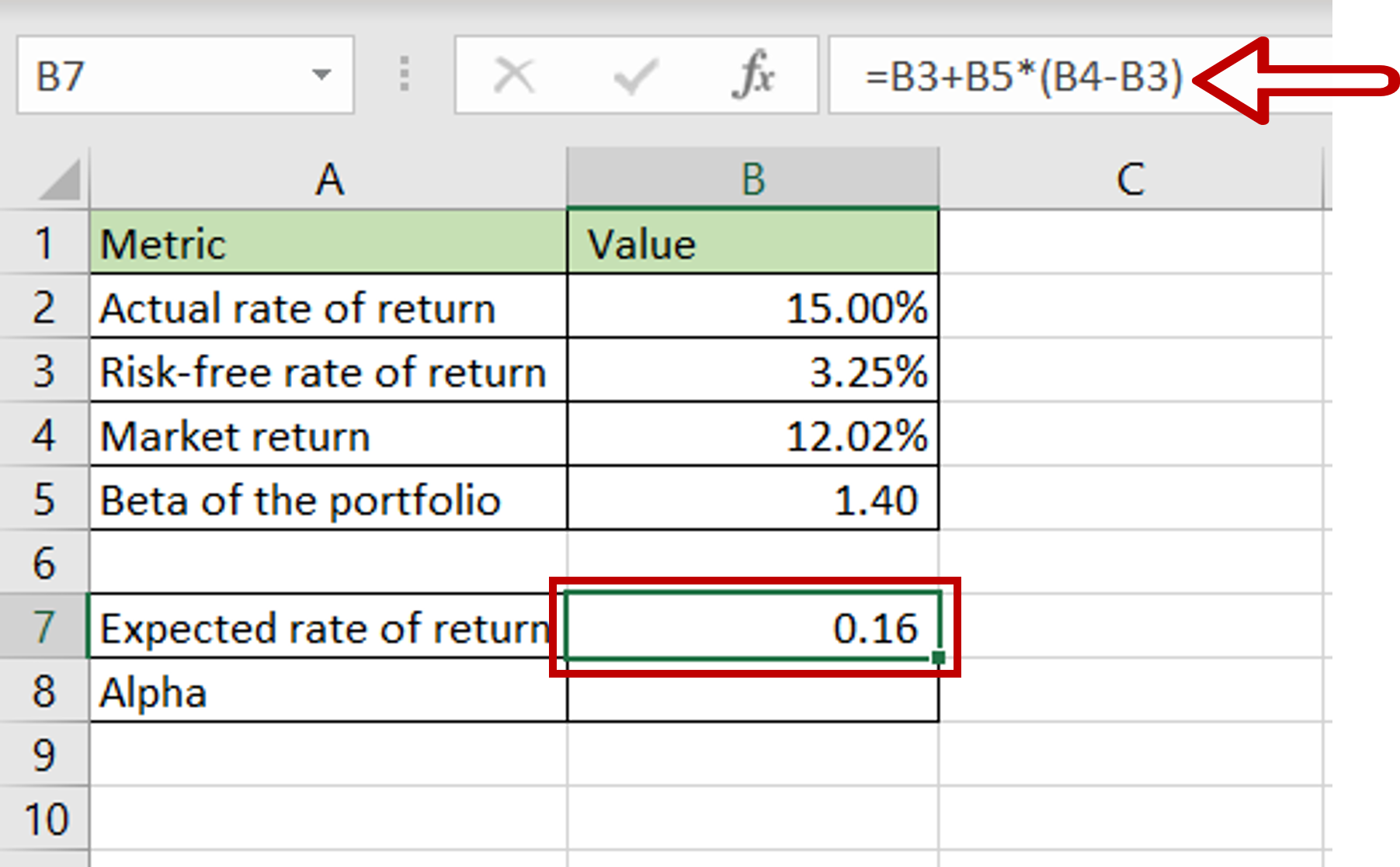# How to calculate alpha in Excel

You can watch a video tutorial here.

To calculate the Alpha in Excel, you need to have the following information in place:

1. Actual rate of return of the portfolio
2. The Risk-free rate of return
3. The Market return
4. Beta of the portfolioAlpha is an important metric used by portfolio managers to measure whether a portfolio has performed better or worse than the market. The formula for calculating the Alpha of a portfolio is:

Alpha = Actual Rate of Return – Expected Rate of Return
> The Actual Rate of Return can be computed based on the actual performance of the portfolio
> Expected Rate of Return = Risk-free rate of return + Beta * (Market return – Risk-free rate of return)

### Step 1 – Compute the Expected Rate of Return– Select the cell where the result is to appear
– Type the formula using cell references:
= Risk-free rate of return + Beta * (Market return – Risk-free rate of return)
– Press Enter

### Step 2 – Convert to percentage– Go to Home > Number
– Click on the percentage (%) button

### Step 3 – Enter the formula for Alpha– Select the cell where the result is to appear
– Type the formula using cell references:
= Actual rate of return – Expected rate of return
– Press Enter
– The Alpha is displayed